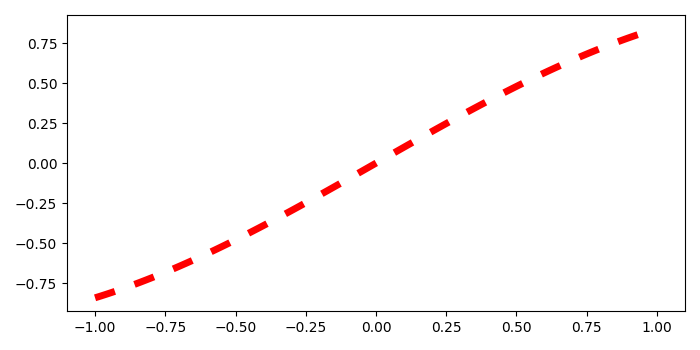# Change the spacing of dashes in a dashed line in Matplotlib?

To change the spacing of dashes in a dashed line in matplotlib, we can take the following steps −

• Create data points x and y using numpy.

• Initialize two variables space and dash_len with value 3.

• Plot x and y using plot() method, with line style '--', dashes tuple stores the property of dashed line.

• To display the figure, use show() method.

## Example

import numpy as np
from matplotlib import pyplot as plt
plt.rcParams["figure.figsize"] = [7.00, 3.50]
plt.rcParams["figure.autolayout"] = True
x = np.linspace(-1, 1, 100)
y = np.sin(x)
space = 3
dash_len = 3
plt.plot(x, y, c='red', linestyle='--', dashes=(dash_len, space), lw=5)
plt.show()

## OutputUpdated on: 08-May-2021

892 Views